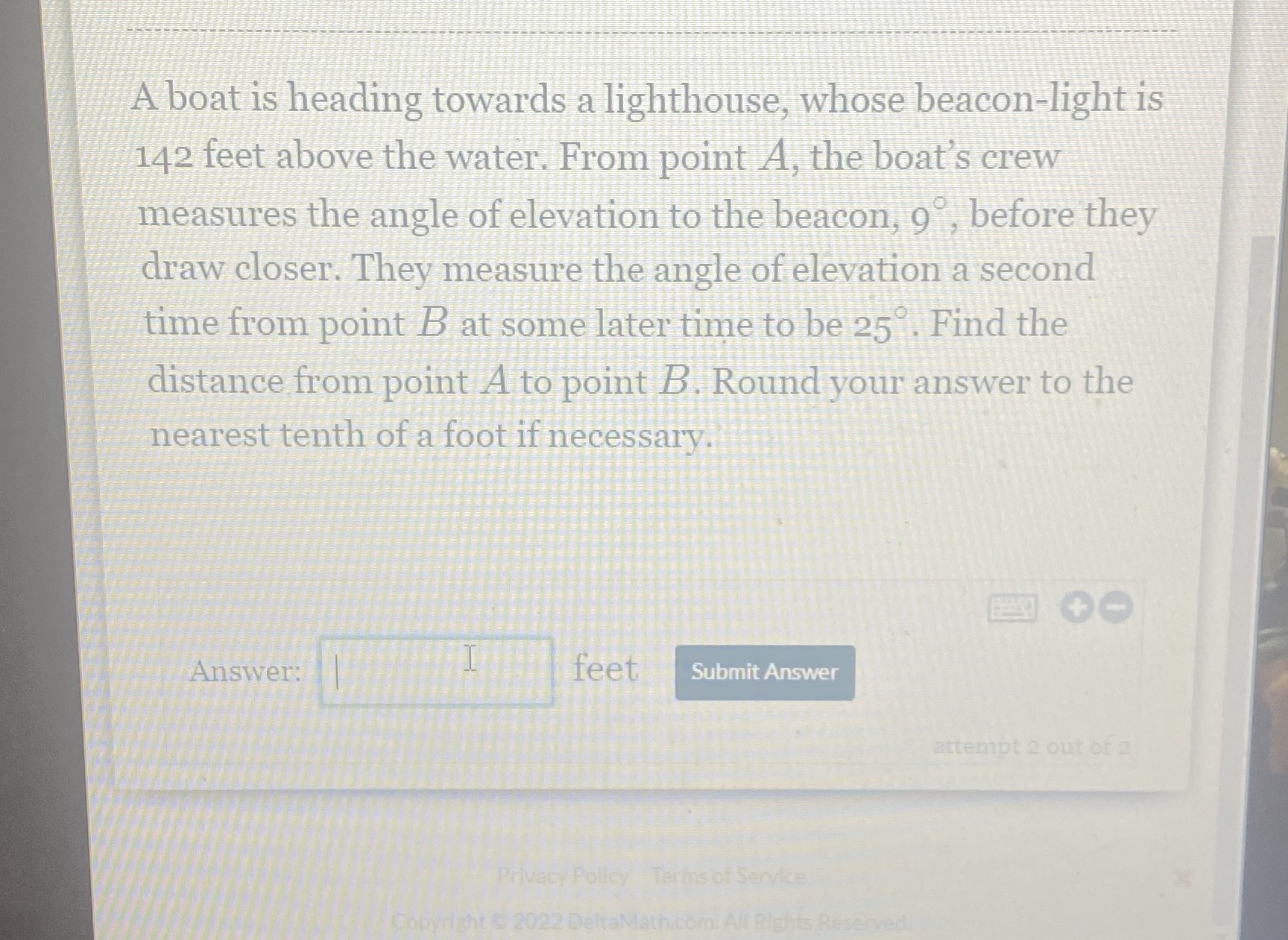### Still have math questions?A boat is heading towards a lighthouse, whose beacon-light is $$142$$ feet above the water. From point $$A$$ , the boat's crew measures the angle of elevation to the beacon, $$9 ^ { \circ }$$ , before they draw closer. They measure the angle of elevation a second time from point $$B$$ at some later time to be $$25 ^ { \circ }$$ . Find the distance from point $$A$$ to point $$B$$ . Round your answer to the nearest tenth of a foot if necessary.